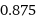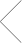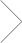## 7/8 as a Decimal Definitions and Examples

7/8 as a Decimal Definitions, Formulas, & Examples

# GET TUTORING NEAR ME!(800) 434-2582

7/8 as a Decimal Definitions and Examples

Introduction

7/8 as a decimal is 0.875. This number can be represented in various ways, including fractions, decimals, and percentages. The way that 7/8 is represented will depend on the context in which it is used. For example, if you were asked to find 7/8 of an inch on a ruler, you would measure 0.875 inches from the left side of the ruler. Or, if you were asked to calculate what 7/8 percent of 100 is, you would multiply 0.875 by 100 to get 87.5. There are many other applications for 7/8 as a decimal, and this blog post will explore some of them in more detail. Keep reading to learn more about this number and how to use it!

7/8 as a Decimal

When we divide 8 by 10, we get 0.8. This is because 8 divided by 10 equals 0 with a remainder of 8. To write this as a decimal, we place the 8 after the decimal point like so: 0.8. So, 8 as a decimal is 0.8.

It’s easy to convert fractions with denominators of 10 into decimals using division. Simply divide the numerator by the denominator and place the result after the decimal point. For example, to convert 2/10 to a decimal, we divide 2 by 10 to get 0.2. We can also convert 6/10 to a decimal by dividing 6 by 10 to get 0.6

What is 7/8 as a decimal?

7/8 as a decimal is .875.

To convert 7/8 to a decimal, divide the numerator by the denominator. In this case, 7 divided by 8 equals .875. Therefore, 7/8 as a decimal is .875.

It’s helpful to know how to convert fractions to decimals because many times numbers are expressed in fraction form when they could be written as a decimal. For example, 3/4 can be written as .75, and 2/3 can be written as .666666… (which rounds to .67). By understanding how to convert fractions like these to decimals, you can more easily work with and compare numbers.

How to convert 7/8 to a decimal

To convert 7/8 to a decimal, divide 7 by 8. The answer is 0.875.

When we divide 7 by 8, we get 0.875. This is because 8 goes into 7 one time, with a remainder of 3. We can write this as a division problem:

7 ÷ 8 = 0 R3

The R stands for remainder. In this case, the 3 is the remainder. When we divide and there is a remainder, we can put the answer in decimal form by moving the decimal point over one place to the left and writing the remainder after the decimal point. So 7 ÷ 8 = 0.875.

7/8 as a decimal point

When we divide 8 by 10, we are essentially asking how many tenths are in 8. The answer, of course, is 0.8 (eight tenths). We can also write this as a decimal using a point, like so: 0.8

This is just one example of how decimals can be used in everyday life. In the real world, we often encounter decimals when dealing with money, measurements, and scientific notation.

Rounding 7/8 as a decimal

When we round 7/8 as a decimal, we are really just finding an estimate for the value of this fraction. To do this, we look at the number to the right of the decimal point and decide whether to round up or down. In this case, we would round down because the number to the right is less than 5. So, our answer would be 0.875.

Estimating with 7/8 as a decimal

7/8 as a decimal is 0.875. To estimate with 7/8 as a decimal, you can round it to the nearest whole number, which would be 1.

Example of 7/8 as a decimal

7/8 as a decimal is .875. This means that 7 out of 8 parts are equal to .875 parts. In other words, if you were to take 8 pieces of something and divide them into 7 equal parts, each part would be .875 of the whole.

Conclusion

The decimal equivalent of 7/8 can be determined by dividing 7 by 8. The answer is 0.875, which means that 7/8 is equal to slightly more than 3/4. This fraction can also be written as a mixed number, with the whole number being 3 and the fractional part being 3/4.

## 7/8 as a Decimal

Decimal formFind the right fit or it’s free.

We guarantee you’ll find the right tutor, or we’ll cover the first hour of your lesson.